# Junction Field Effect Transistor Working?

In general, different types of electrical and electronic components such as transistors, integrated circuits, microcontrollers, transformers, regulators, motors, interfacing devices, modules, and basic components are used (as per requirement) to design different electrical and electronics projects. It is essential to know about the working of each component before using it practically in circuit applications. It is very challenging to discuss in detail about all the important components of electronics in a single article. Hence, let us discuss in detail about junction field effect transistor, JFET characteristics, and its working. But, primarily we must know what are field effect transistors.

### Field Effect Transistors

In solid state electronics, a revolutionary change was done with the invention of the transistor, and is obtained from the words transfer resistor. From the name itself, we can understand the way of functioning of transistor i.e., transfer resistor. The transistors are classified into different types such as a field effect transistor, bipolar junction transistor, and so on.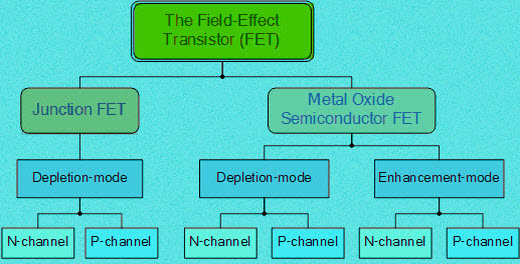Field Effect Transistors

Field effect transistors (FETs) are usually termed as unipolar transistors because these FETs operations are involved with single-carrier type. The field effect transistors are categorized into different types such as a MOSFET, JFET, DGMOSFET, FREDFET, HIGFET, QFET, and so on. But, only MOSFETs (Metal Oxide Semiconductor Field Effect Transistors) and JFETs (Junction Field Effect Transistors) are typically used in most of the applications. So, before discussing in detail about junction field effect transistor, primarily we must know what is JFET.

## Junction Field Effect Transistor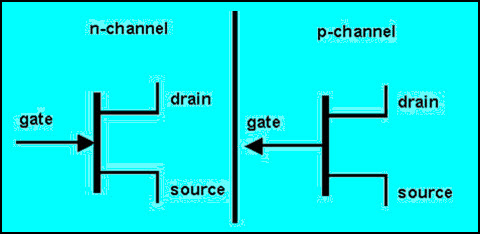Junction Field Effect Transistor

As we discussed earlier, junction field effect transistor is one type of FETs which is used as a switch that can be controlled electrically. Through the active channel, electric energy will flow from between the source terminal and drain terminal. If the gate terminal is supplied with reverse bias voltage, then the flow of current will be completely switched off and the channel gets strained. The junction field effect transistor is generally classified into two types based on their polarities and they are:

• N-Channel junction field effect transistor
• P-Channel junction field effect transistor

#### N-Channel Junction Field Effect Transistor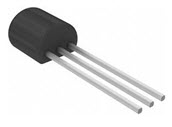N-Channel JFET

The JFET in which electrons are primarily composed as the charge carrier is termed as N-channel JFET. Hence, if the transistor is turned on, then we can say that the current flow is primarily because of the movement of electrons.

#### P-Channel Junction Field Effect Transistor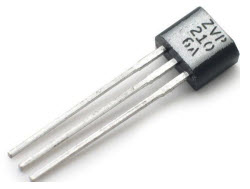P-Channel JFET

The JFET in which holes are primarily composed as the charge carrier is termed as P-channel JFET. Hence, if the transistor is turned on, then we can say that the current flow is primarily because of the holes.

### Working of JFET

Operation of JFET can be studied separately for both N-channel and P-channel.

#### N-Channel Operation of JFET

The working of JFET can be explained by discussing about how to turn on N-channel JFET and how to turn off N-channel JFET. For turning ON a N-channel JFET, positive voltage of VDD has to be applied to the drain terminal of the transistor w.r.t (with respect to) source terminal such that the drain terminal must be appropriately more positive than the source terminal. Thus, current flow is allowed through the drain to source channel. If the voltage at the gate terminal, VGG is 0V, then there will be maximum current at the drain terminal and N-channel JFET is said to be in ON condition.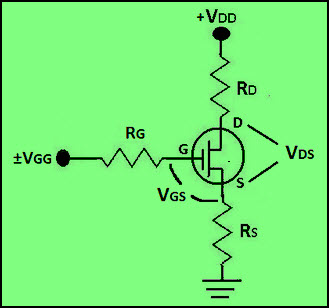N-Channel Operation of JFET

For turning off the N-channel JFET, the positive bias voltage can be turned off or a negative voltage can be applied to the gate terminal. Thus, by changing the polarity of the gate voltage the drain current can be reduced and then N-channel JFET is said to be in OFF condition.

#### P-Channel Operation of JFET

For turning ON P-channel JFET, negative voltage can be applied across the drain terminal of the transistor w.r.t source terminal such that the drain terminal must be appropriately more negative than the source terminal. Thus, the current flow is allowed through the drain to source channel. If the voltage at the gate terminal, VGG is 0V, then there will be maximum current at the drain terminal and the P-channel JFET is said to be in ON condition.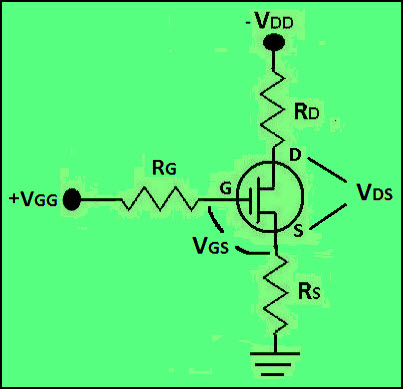P-Channel Operation of JFET

For turning OFF the P-channel JFET, the negative bias voltage can be turned off or positive voltage can be applied to the gate terminal. If the gate terminal is given positive voltage, then the drain currents starts reducing (until cutoff) and thus the P-channel JFET is said to be in OFF condition.

### JFET Characteristics

The JFET characteristics of can be studied for both N-channel and P-channel as discussed below:

#### N-Channel JFET Characteristics

The N-channel JFET characteristics or transconductance curve is shown in the figure below which is graphed between drain current and gate-source voltage. There are multiple regions in the transconductance curve and they are ohmic, saturation, cutoff, and breakdown regions.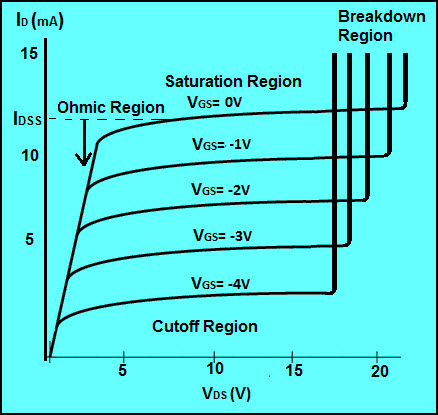N-Channel JFET Characteristics

Ohmic Region
The only region in which transconductance curve shows linear response and drain current is opposed by the JFET transistor resistance is termed as Ohmic region.
Saturation Region
In the saturation region, the N-channel junction field effect transistor is in ON condition and active, as maximum current flows because of the gate-source voltage applied.
Cutoff Region
In this cutoff region, there will be no drain current flowing and thus, the N-channel JFET is in OFF condition.
Breakdown Region
If the VDD voltage applied to the drain terminal exceeds the maximum necessary voltage, then the transistor fails to resist the current and thus, the current flows from drain terminal to source terminal. Hence, the transistor enters into the breakdown region.

#### P-Channel JFET Characteristics

The P-channel JFET characteristics or transconductance curve is shown in the figure below which is graphed between drain current and gate-source voltage. There are multiple regions in the transconductance curve and they are ohmic, saturation, cutoff, and breakdown regions.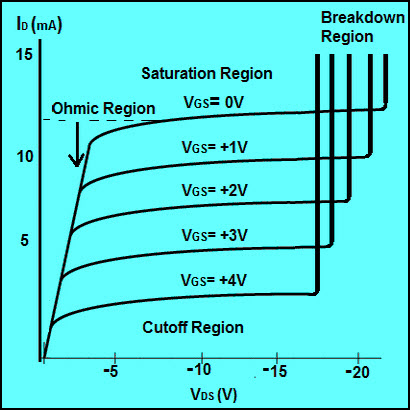P-Channel JFET Characteristics

Ohmic Region
The only region in which transconductance curve shows linear response and drain current is opposed by the JFET transistor resistance is termed as Ohmic region.
Saturation Region
In the saturation region, the N-channel junction field effect transistor is in ON condition and active, as maximum current flows because of the gate-source voltage applied.
Cutoff Region
In this cutoff region, there will be no drain current flowing and thus, the N-channel JFET is in OFF condition.
Breakdown Region
If the VDD voltage applied to the drain terminal exceeds the maximum necessary voltage, then the transistor fails to resist the current and thus, the current will flow from drain terminal to source terminal. Hence, the transistor enters into the breakdown region.

Do you want to know the practical applications of junction field effect transistor in designing electronics projects? Then, post your comments in the comments section below for further technical assistance.

## 2 Comments

1. Nishu yadav says:

Define all types of transister